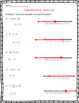# 7th Grade Math – Expressions, Equations and Inequalities BUNDLESubject
Resource Type
File Type

Zip

(9 MB|274 pages)
Standards
• Product Description
• Standards

7th Grade Math – Expressions, Equations and Inequalities Bundle

This is a bundle of lessons that target the Common Core Math Standards associated with Algebraic Expressions, Equations and Inequalities.

What’s included?

• Lesson 1- Writing Algebraic Expressions

• Lesson 2 – Simplifying Algebraic Expressions

• Lesson 3 – One Step Equations

• Lesson 4 – Two Step Equations

• Lesson 5 – One Step Inequalities

• Lesson 6 – Two Step Inequalities

• Quiz 1 – Expressions and Equations

• Quiz 2 – Inequalities

• Summative Assessment

***We also have the entire 7th grade Curriculum available here: ***

7th Grade Math Bundle – Year Long Curriculum

****************************************************************************************************************************************************

7th Grade Math – Expressions, Equations and Inequalities Unit

Each Lesson Includes:

• Lesson Plan

• Power Point Presentation

• Warm Up Activity

• Guided Notes

• Cooperative Learning Activity

• Independent Practice

• Formative Assessment

****************************************************************************************************************************************************

Thanks,

Lessons for Middle School

Solve word problems leading to inequalities of the form 𝘱𝘹 + 𝘲 > 𝘳 or 𝘱𝘹 + 𝘲 < 𝘳, where 𝘱, 𝘲, and 𝘳 are specific rational numbers. Graph the solution set of the inequality and interpret it in the context of the problem. For example: As a salesperson, you are paid \$50 per week plus \$3 per sale. This week you want your pay to be at least \$100. Write an inequality for the number of sales you need to make, and describe the solutions.
Solve word problems leading to equations of the form 𝘱𝘹 + 𝘲 = 𝘳 and 𝘱(𝘹 + 𝘲) = 𝘳, where 𝘱, 𝘲, and 𝘳 are specific rational numbers. Solve equations of these forms fluently. Compare an algebraic solution to an arithmetic solution, identifying the sequence of the operations used in each approach. For example, the perimeter of a rectangle is 54 cm. Its length is 6 cm. What is its width?
Use variables to represent quantities in a real-world or mathematical problem, and construct simple equations and inequalities to solve problems by reasoning about the quantities.
Solve multi-step real-life and mathematical problems posed with positive and negative rational numbers in any form (whole numbers, fractions, and decimals), using tools strategically. Apply properties of operations to calculate with numbers in any form; convert between forms as appropriate; and assess the reasonableness of answers using mental computation and estimation strategies. For example: If a woman making \$25 an hour gets a 10% raise, she will make an additional 1/10 of her salary an hour, or \$2.50, for a new salary of \$27.50. If you want to place a towel bar 9 3/4 inches long in the center of a door that is 27 1/2 inches wide, you will need to place the bar about 9 inches from each edge; this estimate can be used as a check on the exact computation.
Understand that rewriting an expression in different forms in a problem context can shed light on the problem and how the quantities in it are related. For example, 𝘢 + 0.05𝘢 = 1.05𝘢 means that “increase by 5%” is the same as “multiply by 1.05.”
Total Pages
274 pages
Included
Teaching Duration
2 Weeks
Report this Resource to TpT
Reported resources will be reviewed by our team. Report this resource to let us know if this resource violates TpT’s content guidelines.